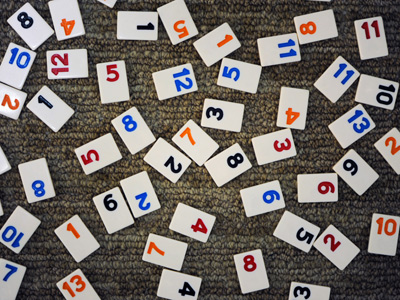987,642 is the largest number that can be made from the digits 2, 4, 6, 7, 8, 9.

# Compare and Order Numbers (Year 5)

One of the most important skills children develop in KS2 Maths is the ability to compare and order numbers. This includes ordering negative numbers, decimal numbers and, by year five, five and six figure numbers, as well as recognising the greater than >, smaller than < and equal to = symbols. This is the second of two quizzes on the subject and it is aimed primarily at children in Year Five.

Comparing and ordering numbers is useful when listing them by size. A good understanding of the value of a figure means you can compare one number with another and put several numbers in order. For example, a six in the hundreds column is worth 600 but in the thousands column it is worth 6,000.

Take this quiz and find out how well you can compare and order decimal, negative or six figure numbers. For 9-10 year olds.

1.
What is the largest number that can be made from the digits 2, 4, 6, 7, 8, 9?
986,742
968,742
978, 624
987,642
9 goes in the hundred thousands column
8 in the ten thousands column
7 in the thousands column
6 in the hundreds,
4 in the tens and
2 in the units
2.
Which is the least amount?
54,697
54,796
54,976
54,967
All have 54 thousands but 54,697 has only 6 hundreds. The other three numbers have at least 7 hundreds
3.
What does the symbol ≥ mean?
Less than or equal to
More than or equal to
Equal to
Not equal to
The line beneath the more than symbol means the number could also be equal to
4.
Which number comes before -12 on a numberline?
-10
-11
-13
-9
-13 is one less than -12
5.
What does the symbol ≤ mean?
Less than or equal to
More than or equal to
Equal to
Not equal to
The arrow points to the smaller number
6.
Which of these sequences is in descending order?
5, 10, 15, 20, 25, 30
2.5, 2.6, 2.7, 2.8, 2.9
4,578, 4,579, 4,580, 4,581
68, 66, 64, 63, 62
Descending order means the numbers are getting smaller
7.
Which of these sequences is in ascending order?
56, 55, 54, 53, 52
455, 450, 445, 440, 435
0.1, 0.2, 0.3, 0.4, 0.5
23, 22, 21, 20, 19, 18
Ascending order means the numbers are getting larger
8.
Which number is halfway between 27,400 and 28,000?
27,500
27,600
27,700
27,800
28,000 - 27,400 = 600
600 ÷ 2 = 300
27,400 + 300 = 27,700
9.
Which number is less than -34?
-32
-35
34
-1
With minus numbers the higher the figure the lower the number
10.
Which of these numbers comes first on a number line?
0.1
0.25
0.125
0.5
The smallest number will always come first on a number line
You can find more about this topic by visiting BBC Bitesize - Order numbers up to 100,000

Author:  Amanda Swift